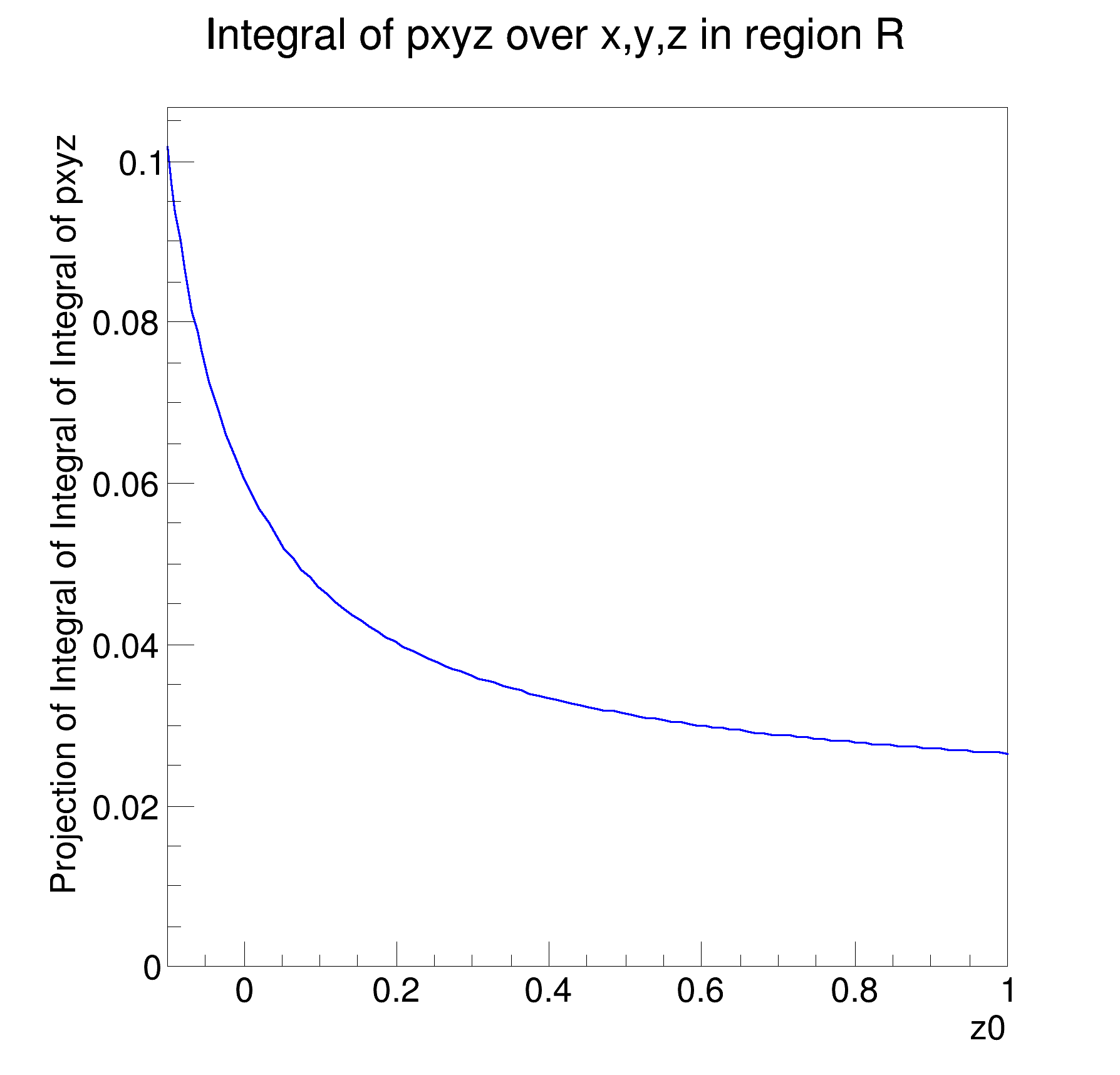ROOT   Reference Guide
Searching...
No Matches
rf313_paramranges.C File Reference

## Detailed DescriptionMultidimensional models: working with parametrized ranges to define non-rectangular regions for fitting and integration␛[1mRooFit v3.60 -- Developed by Wouter Verkerke and David Kirkby␛[0m
Copyright (C) 2000-2013 NIKHEF, University of California & Stanford University
[#1] INFO:NumericIntegration -- RooRealIntegral::init(pxyz_Int[z|R]_Norm[x,y,z]_Int[y|R]) using numeric integrator RooIntegrator1D to calculate Int(y)
[#1] INFO:NumericIntegration -- RooRealIntegral::init(pxyz_Int[z|R]_Norm[x,y,z]_Int[y|R]_Int[x|R]) using numeric integrator RooIntegrator1D to calculate Int(x)
[#1] INFO:NumericIntegration -- RooRealIntegral::init(pxyz_Int[z|R]_Norm[x,y,z]_Int[y|R]) using numeric integrator RooIntegrator1D to calculate Int(y)
#include "RooRealVar.h"
#include "RooDataSet.h"
#include "RooGaussian.h"
#include "RooConstVar.h"
#include "RooPolynomial.h"
#include "RooProdPdf.h"
#include "TCanvas.h"
#include "TAxis.h"
#include "RooPlot.h"
using namespace RooFit;
{
// C r e a t e 3 D p d f
// -------------------------
// Define observable (x,y,z)
RooRealVar x("x", "x", 0, 10);
RooRealVar y("y", "y", 0, 10);
RooRealVar z("z", "z", 0, 10);
// Define 3 dimensional pdf
RooRealVar z0("z0", "z0", -0.1, 1);
RooPolynomial px("px", "px", x, RooConst(0));
RooPolynomial py("py", "py", y, RooConst(0));
RooPolynomial pz("pz", "pz", z, z0);
RooProdPdf pxyz("pxyz", "pxyz", RooArgSet(px, py, pz));
// D e f i n e d n o n - r e c t a n g u l a r r e g i o n R i n ( x , y , z )
// -------------------------------------------------------------------------------------
//
// R = Z[0 - 0.1*Y^2] * Y[0.1*X - 0.9*X] * X[0 - 10]
//
// Construct range parametrized in "R" in y [ 0.1*x, 0.9*x ]
RooFormulaVar ylo("ylo", "0.1*x", x);
RooFormulaVar yhi("yhi", "0.9*x", x);
y.setRange("R", ylo, yhi);
// Construct parametrized ranged "R" in z [ 0, 0.1*y^2 ]
RooFormulaVar zlo("zlo", "0.0*y", y);
RooFormulaVar zhi("zhi", "0.1*y*y", y);
z.setRange("R", zlo, zhi);
// C a l c u l a t e i n t e g r a l o f n o r m a l i z e d p d f i n R
// ----------------------------------------------------------------------------------
// Create integral over normalized pdf model over x,y,z in "R" region
RooAbsReal *intPdf = pxyz.createIntegral(RooArgSet(x, y, z), RooArgSet(x, y, z), "R");
// Plot value of integral as function of pdf parameter z0
RooPlot *frame = z0.frame(Title("Integral of pxyz over x,y,z in region R"));
intPdf->plotOn(frame);
new TCanvas("rf313_paramranges", "rf313_paramranges", 600, 600);
frame->GetYaxis()->SetTitleOffset(1.6);
frame->Draw();
return;
}
RooAbsReal is the common abstract base class for objects that represent a real value and implements f...
Definition RooAbsReal.h:61
virtual RooPlot * plotOn(RooPlot *frame, const RooCmdArg &arg1=RooCmdArg(), const RooCmdArg &arg2=RooCmdArg(), const RooCmdArg &arg3=RooCmdArg(), const RooCmdArg &arg4=RooCmdArg(), const RooCmdArg &arg5=RooCmdArg(), const RooCmdArg &arg6=RooCmdArg(), const RooCmdArg &arg7=RooCmdArg(), const RooCmdArg &arg8=RooCmdArg(), const RooCmdArg &arg9=RooCmdArg(), const RooCmdArg &arg10=RooCmdArg()) const
Plot (project) PDF on specified frame.
RooAbsReal * createIntegral(const RooArgSet &iset, const RooCmdArg &arg1, const RooCmdArg &arg2=RooCmdArg::none(), const RooCmdArg &arg3=RooCmdArg::none(), const RooCmdArg &arg4=RooCmdArg::none(), const RooCmdArg &arg5=RooCmdArg::none(), const RooCmdArg &arg6=RooCmdArg::none(), const RooCmdArg &arg7=RooCmdArg::none(), const RooCmdArg &arg8=RooCmdArg::none()) const
Create an object that represents the integral of the function over one or more observables listed in ...
RooArgSet is a container object that can hold multiple RooAbsArg objects.
Definition RooArgSet.h:29
A RooFormulaVar is a generic implementation of a real-valued object, which takes a RooArgList of serv...
A RooPlot is a plot frame and a container for graphics objects within that frame.
Definition RooPlot.h:44
TAxis * GetYaxis() const
Definition RooPlot.cxx:1263
static RooPlot * frame(const RooAbsRealLValue &var, Double_t xmin, Double_t xmax, Int_t nBins)
Create a new frame for a given variable in x.
Definition RooPlot.cxx:249
virtual void Draw(Option_t *options=0)
Draw this plot and all of the elements it contains.
Definition RooPlot.cxx:691
RooPolynomial implements a polynomial p.d.f of the form.
RooProdPdf is an efficient implementation of a product of PDFs of the form.
Definition RooProdPdf.h:37
RooRealVar represents a variable that can be changed from the outside.
Definition RooRealVar.h:39
virtual void SetTitleOffset(Float_t offset=1)
Set distance between the axis and the axis title.
Definition TAttAxis.cxx:293
The Canvas class.
Definition TCanvas.h:23
Double_t y[n]
Definition legend1.C:17
Double_t x[n]
Definition legend1.C:17
The namespace RooFit contains mostly switches that change the behaviour of functions of PDFs (or othe...
Date
July 2008

Definition in file rf313_paramranges.C.